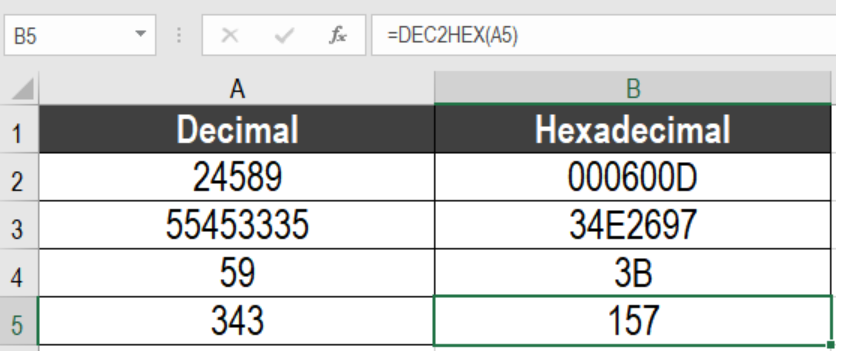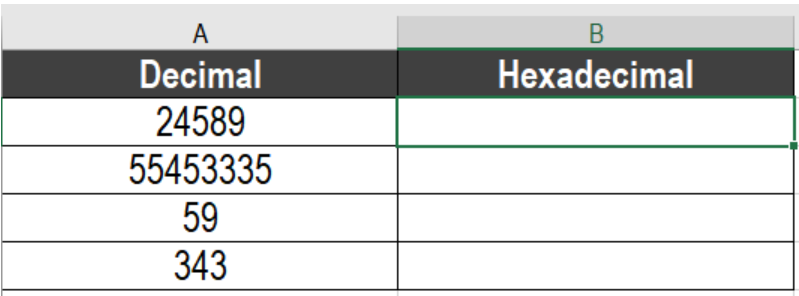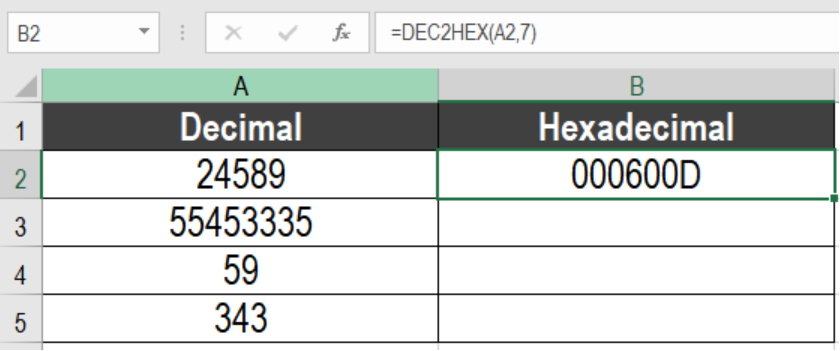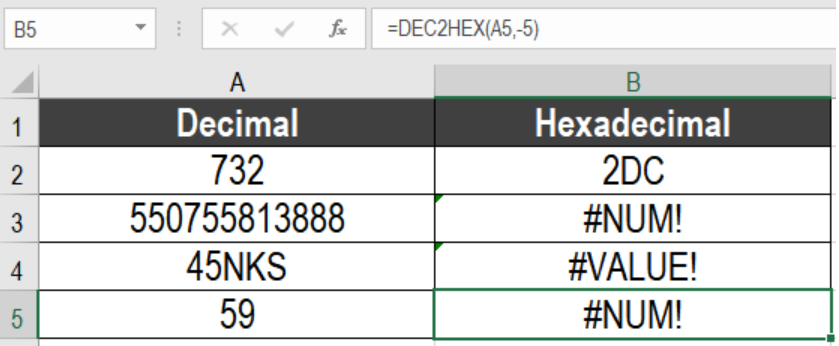Get instant live expert help with Excel or Google Sheets“My Excelchat expert helped me in less than 20 minutes, saving me what would have been 5 hours of work!”

#### Post your problem and you'll get expert help in seconds

Your message must be at least 40 characters
Our professional experts are available now. Your privacy is guaranteed.

# How to use the Excel DEC2HEX Function

We have some very effective functions when it comes to working with numbers in Excel. DEC2HEX is one such function. It takes a decimal number and converts it to its hexadecimal counterpart. In this tutorial, we will learn how to use the DEX2HEC function in Excel.Figure 1. Example of How to Use the DEC2HEX Function in Excel

## Syntax for DEC2HEX Function

`=DEC2HEX(number, [places])`

• Number: Required. This is the decimal number to convert to its hexadecimal equivalent.
• [places]: Optional. Rounds up the output number with zeros up to the digits specified. If we omit it, DEC2HEX returns the minimum number of characters needed to show the number.

## Setting up Data

The following data set has some sample decimal numbers. Column A contains the numbers.

Figure 2. The Sample Data SetTo convert these numbers to hexadecimal in column B:

• We need to click cell B2 with the mouse.
• Assign the formula `=DEC2HEX(A2,7)` to the formula bar of cell B2.
• Press Enter.

Figure 3. Example of Applying the DEC2HEX Function• Assign the formula `=DEC2HEX(A3)` to cell B3. Press Enter and drag the fill handle from cells B3 to B5.

Column B will now have the hexadecimal representation of the decimal numbers in column A. Cell B2 has the formula with the places argument 7. This rounds up the output 600D with 0’s in the first three digits.

## Notes

• The DEC2HEX function requires a valid input of a decimal number within the range [ -2^39, 2^39 – 1]. Otherwise it returns a #NUM! error.
• DEC2HEX returns a #VALUE! error if the number is non numeric.
• It returns a #NUM! Error if the result of DEC2HEX requires more numbers than the places argument.
• Excel truncates the value of the places argument if it is not an integer.
• DEC2HEX returns a #VALUE! error if places is non numeric. It returns a #NUM! error if places is negative.Figure 4. Example of Common Errors of the DEC2HEX Function

Most of the time, the problem you will need to solve will be more complex than a simple application of a formula or function. If you want to save hours of research and frustration, try our live Excelchat service! Our Excel Experts are available 24/7 to answer any Excel question you may have. We guarantee a connection within 30 seconds and a customized solution within 20 minutes.